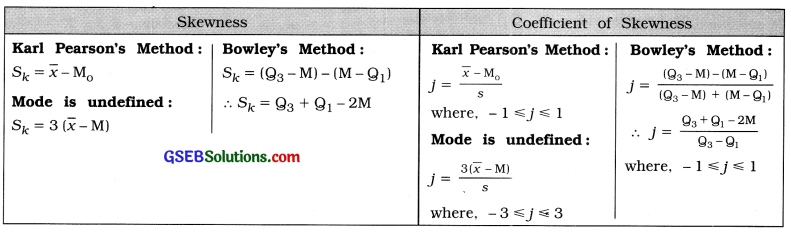# GSEB Class 11 Statistics Notes Chapter 5 Skewness of Frequency Distribution

This GSEB Class 11 Commerce Statistics Notes Chapter 5 Skewness of Frequency Distribution covers all the important topics and concepts as mentioned in the chapter.

## Skewness of Frequency Distribution Class 11 GSEB Notes

Meaning of Skewness:
Symmetric Frequency Distribution:
A frequency distribution in which the observations of the data are evenly distributed on both the sides of mode is called symmetric frequency distribution. The frequency curve of the symmetric frequency distribution is found to be bell-shaped.

Characteristics of Symmetric Frequency Distribution:

• Its frequency curve is bell-shaped.
• The values of mean, median and mode are equal, i.e., x̄ = M = M0.
• Quartiles Q1 and Q3 are equidistant from median M, i.e., (Q3 – M) = (M – Q1), and
• The frequency of observation at equal distance on the both sides of mode is evenly distributed.

Skewed Frequency Distribution:
The frequency distribution with lack of symmetry is called skewed frequency distribution.

Skewness:
The lack of symmetry is called skewness.

Tests of Skewness:

• The frequency curve is not bell-shaped.
• The values of mean, median and mode are not equal, i. e., x ≠ M ≠ M0.
• Quartiles Q1 and Q3 are not equidistant from median M. i.e., (Q3 – M) ≠ (M – Q1), and
• The frequency of observation at equidistant on both the sides of more is not evenly distributed.Types of Skewness:
Positive Skewness:

• The right tail of the frequency curve more elongated
• x̄ > M > M0 and
• (Q3 – M) > (M – Q1).

Negative Skewness:

• The left tail of the frequency curve is more enlogated
• x̄ < M < M0 and
• (Q3 – M) < (M – Q1).

Types of Measures of Skewness:
Absolute Measure:
A measure of skewness which is expressed in the same units of observations of the data is called an absolute measure of skewness usually, it is called ‘Skewness’. It is denoted by Sk.

• It is not useful for comparing skewness of two or more frequency distribution.
• Even of the units of two or more population are same, it is not advisable to compare them using skewness.

Relative Measure:
A measure of skewness which is free from the unit of measurement is called the relative measure of skewness. It is called coefficient of skewness. It is denoted by ‘J’. It is obtained by dividing the absolute measure by an appropriate measure of dispersion.

• It is free from unit and hence it is used for comperative study of two or more population.
• Its value may be zero, positive or negative. Usually the range of its value is – 1 to 1. But in special circumstances, the range of its value may be -3 to 3.

Methods of obtaining Measures of Skewness:
1. Karl Pearson’s Method:
Base: In a skewed frequency distribution the values of mean, median and mode are not equal.

Formula:
(i) For unimodal frequency distribution:
It is obtained by taking the difference of mean and mode.
Sk = x̄ – M0 and j = $$\frac{\bar{x}-M_{o}}{s}$$; 1 ≤ j ≤ 1
Where, x = Mean; M0= Mode; s = standard deviation.

(ii) Mode is not defined in frequency distribution:
Sk = 3 (x̄ – M) and j= $$\frac{3(\bar{x}-\mathrm{M})}{\mathrm{s}}$$, -3 ≤ j ≤ 3

2. Bowley’s Method:
Base:
In a skewed frequency distribution quartiles Q1 and Q3 are not equidistant from median M.

Formula:
It is obtained by taking the difference of distances of Q3 from M and M from Q1.
Sk = (Q3 – M) – (M – Q1)
= Q3 + Q1 – 2M and
= (Q3 – M) -(M – Q1)

j = $$=\frac{\left(Q_{3}-M\right)-\left(M-Q_{1}\right)}{\left(Q_{3}-M\right)+\left(M-Q_{1}\right)}$$
= $$\frac{Q_{3}+Q_{1}-2 M}{Q_{3}-Q_{1}}$$, -1 ≤ j ≤ 1Comparison of Two Methods of Finding Coefficient of Skewness:

• Bowley’s method is easier in calculation than Karl Pearson’s method.
• The value of coefficient of skewness obtained by Karl Pearson’s method is more reliable than by Bowley’s method.
• Bowley’s method is the only method used for finding the coefficient of skewness for the frequency distribution with open-ended classes.
• It is inappropriate to compare the values obtained by both methods as the sign of the coefficient of skewness may not be same.
• If the coefficient of skewness j = 0, it is possible in some cases that the frequency distribution has positive or negative skewness.

Important Formulae:
Discret Frequency Distribution and Continuous Frequency Distribution:Remember for calculating Coefficient of Skewness

• When it is not given that by which method coefficient of skewness is to be found, use Karl Pearson’s method.
• If given frequency distribution is open-ended, use Bowley’s method to find the coefficient of skewness.
• If the Mode is undefined for the given frequency distribution, that is
1. The frequency distribution has unequal class length,
2. The frequency distribution is of mixed type
3. The highest frequency lies against two or more class intervals, find the value of coefficient of skewness by using Karl Pearson s formula j = $$\frac{3(\bar{x}-\mathrm{M})}{\mathrm{s}}$$
• If class intervals of the frequency distribution are not continuous, obtain boundary points of related classes to find the Median, Mode and Quartiles.
• If the given frequency distribution is ‘less than’ or ‘more than’ type of cumulative frequency distributions, first obtain original frequency distribution. Keep it in mind that the cumulative frequencies are given in such type of frequency distribution.
• When mid values are given despite class intervals, obtain boundary points of class for each mid value by the following formulae to find the Mean, Median and Quartiles:
• Lower boundary point = Mid Value – $$\frac{\text { class interval }}{2}$$
• Upper boundary point = Mid value + $$\frac{\text { class interval }}{2}$$
• Always write observations or classes in ascending orders of their magnitudes.
• The value of coefficient of skewness can be positive, negative or zero.